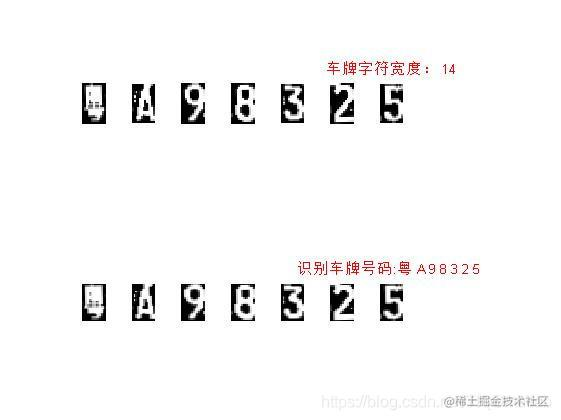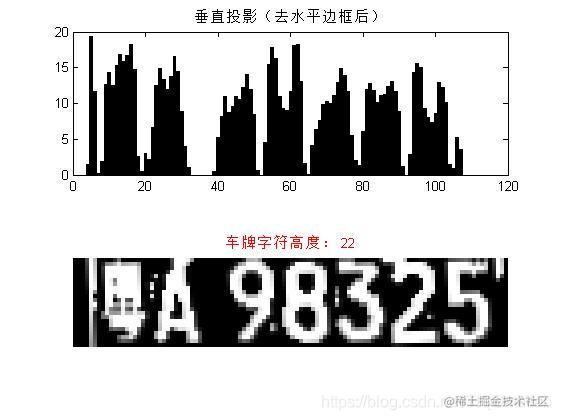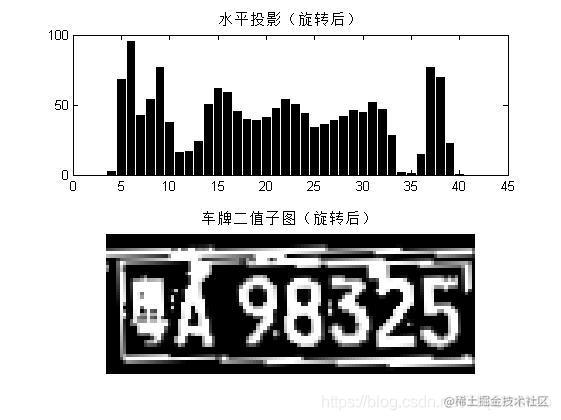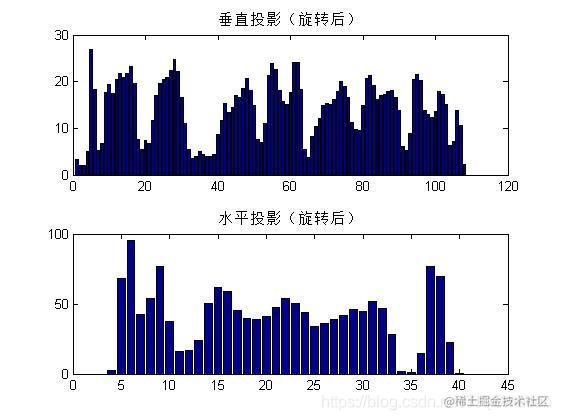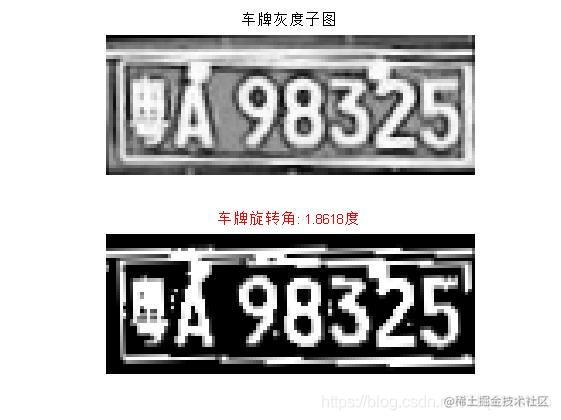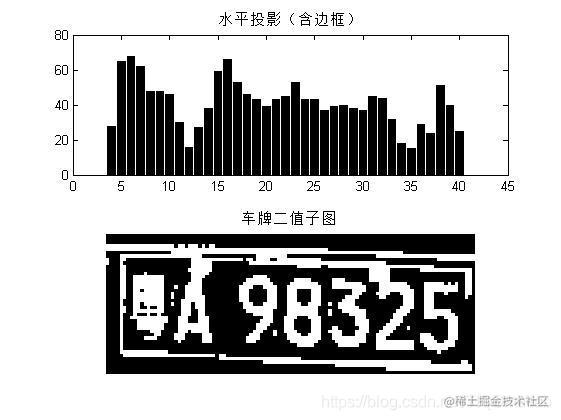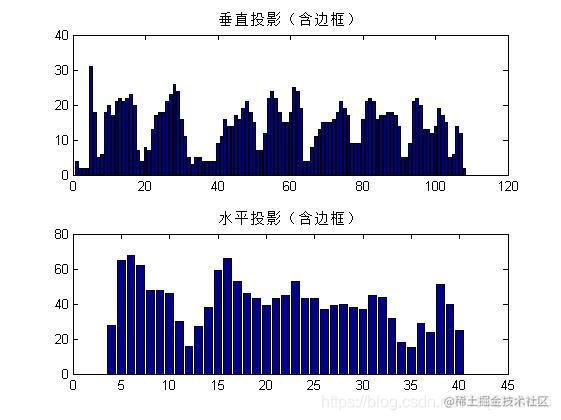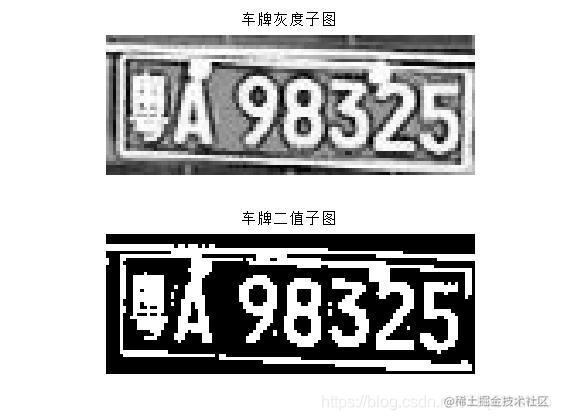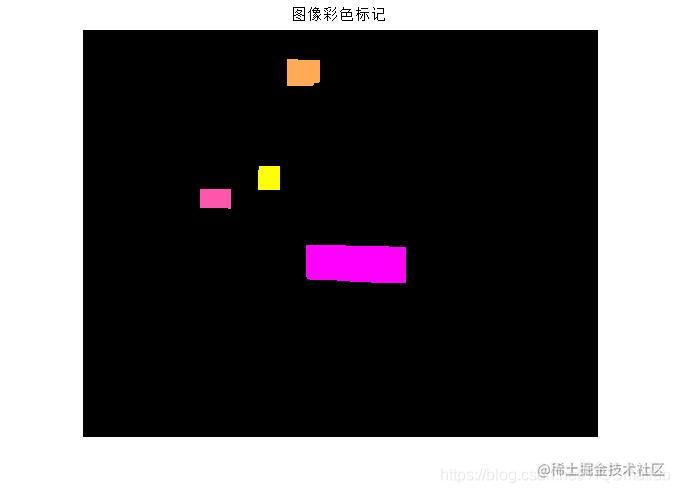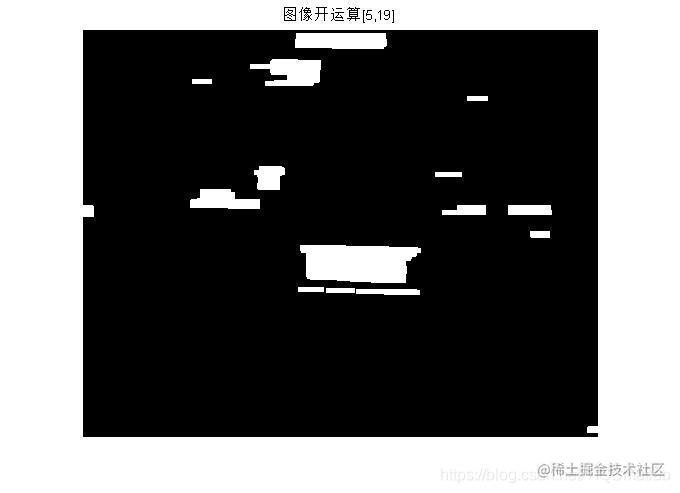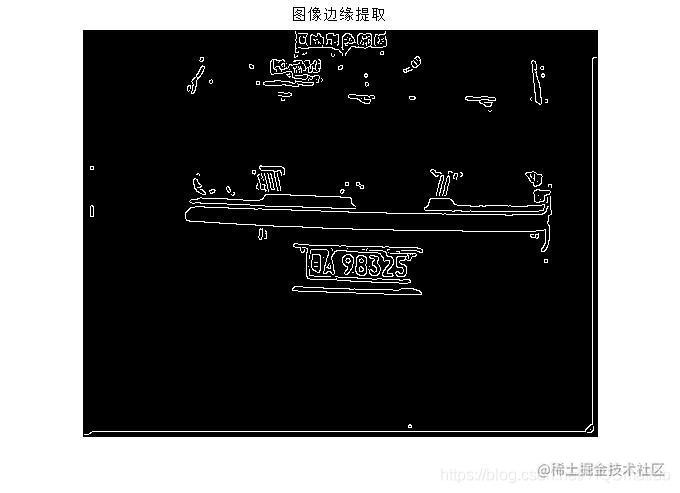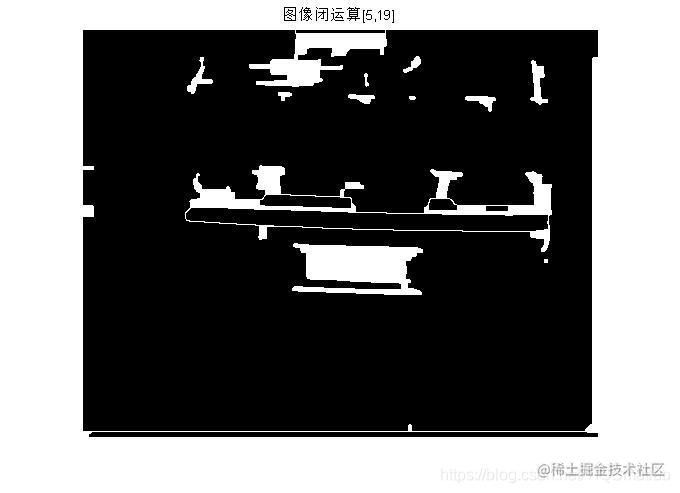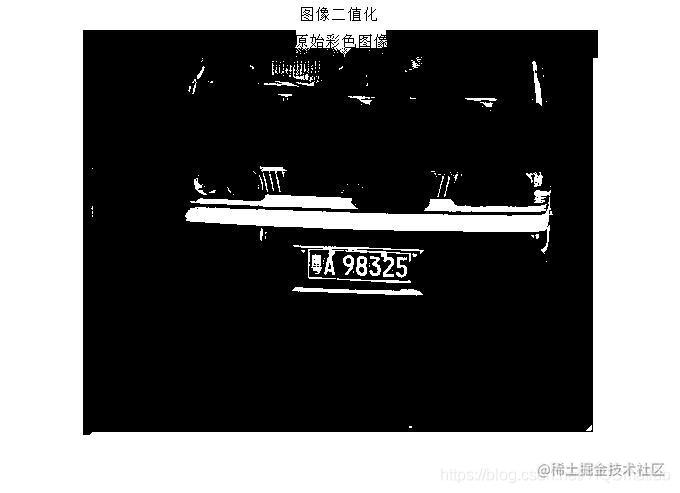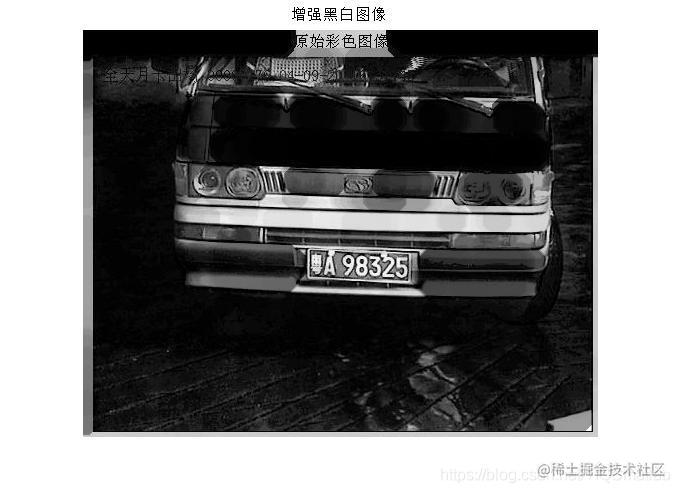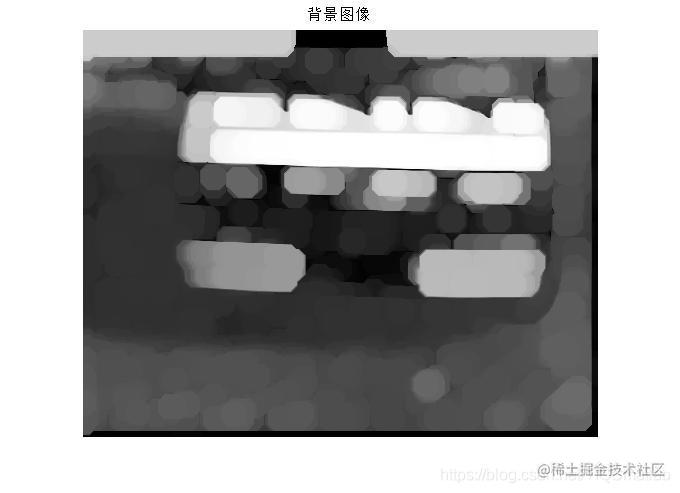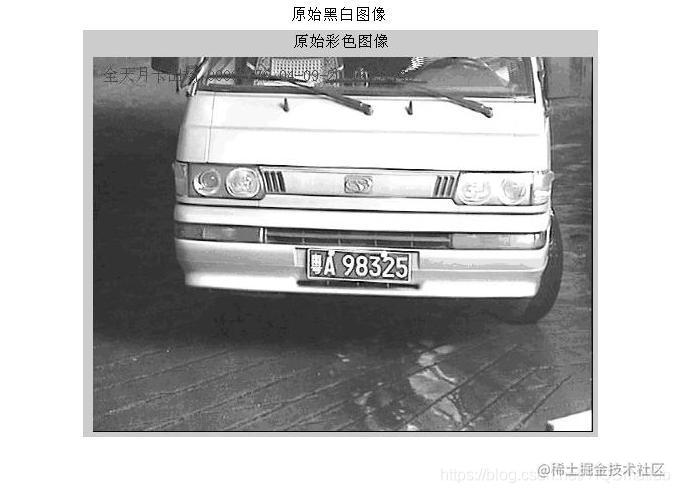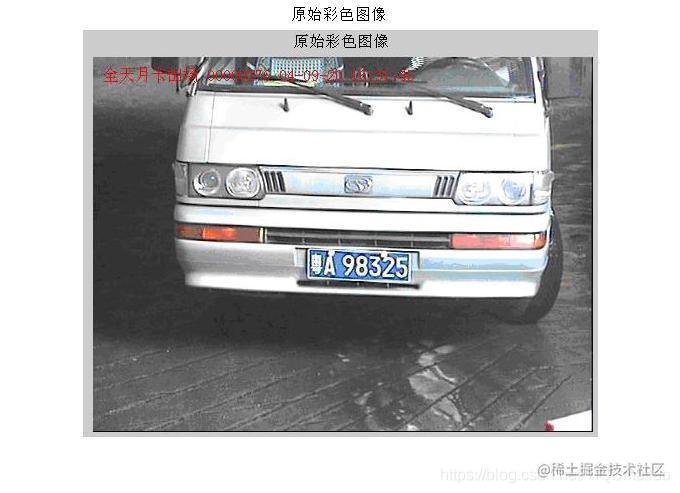# 【车牌识别】基于matlab形态学车牌识别【含Matlab源码 1155期】

## 一、简介

1 摘要 随这图形图像技术的发展，现在的车牌识别技术准确率越来越高，识别速度越来越快。无论何种形式的车牌识别系统，它们都是由触发、图像采集、图像识别模块、辅助光源和通信模块组成的。车牌识别系统涉及光学、电器、电子控制、数字图像处理、计算视觉、人工智能等多项技术。触发模块负责在车辆到达合适位置时，给出触发信号，控制抓拍。辅助光源提供辅助照明，保证系统在不同的光照条件下都能拍摄到高质量的图像。图像预处理程序对抓拍的图像进行处理，去除噪声，并进行参数调整。然后通过车牌定位、字符识别，最后将识别结果输出。

2 设计目的和意义 2.1设计目的： 2.1.1让学生巩固理论课上所学的知识，理论联系实践。 2.1.2 锻炼学生的动手能力，激发学生的研究潜能，提高学生的协作精神。 2.2 设计意义： 车牌定位系统的目的在于正确获取整个图像中车牌的区域，并识别出车牌号。通过设计实现车牌识别系统，能够提高学生分析问题和解决问题的能力，还能培养一定的科研能力。

3 设计原理 牌照自动识别是一项利用车辆的动态视频或静态图像进行牌照号码、牌照颜色自动识别的模式识别技术。其硬件基础一般包括触发设备、摄像设备、照明设备、图像采集设备、识别车牌号码的处理机等，其软件核心包括车牌定位算法、车牌字符分割算法和光学字符识别算法等。某些牌照识别系统还具有通过视频图像判断车辆驶入视野的功能称之为视频车辆检测。一个完整的牌照识别系统应包括车辆检测、图像采集、牌照识别等几部分。当车辆检测部分检测到车辆到达时触发图像采集单元，采集当前的视频图像。牌照识别单元对图像进行处理，定位出牌照位置，再将牌照中的字符分割出来进行识别，然后组成牌照号码输出。

4 详细设计步骤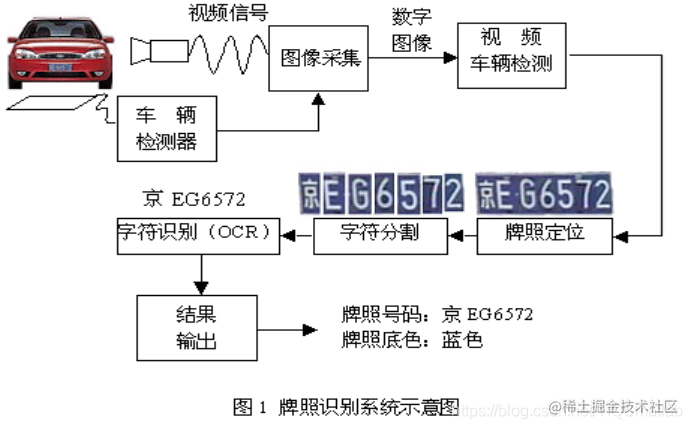1. 提出总体设计方案:

## 二、源代码

``````clear ;
close all;

%Step1 获取图像   装入待处理彩色图像并显示原始图像
%将彩色图像转换为黑白并显示
Sgray = rgb2gray(Scolor);%rgb2gray转换成灰度图
figure,imshow(Scolor),title('原始彩色图像');%figure命令同时显示两幅图像
figure,imshow(Sgray),title('原始黑白图像');
%Step2 图像预处理   对Sgray 原始黑白图像进行开操作得到图像背景
s=strel('disk',13);%strei函数
Bgray=imopen(Sgray,s);%打开sgray s图像
figure,imshow(Bgray);title('背景图像');%输出背景图像
%用原始图像与背景图像作减法，增强图像
Egray=imsubtract(Sgray,Bgray);%两幅图相减
figure,imshow(Egray);title('增强黑白图像');%输出黑白图像
%Step3 取得最佳阈值，将图像二值化
fmax1=double(max(max(Egray)));%egray的最大值并输出双精度型
fmin1=double(min(min(Egray)));%egray的最小值并输出双精度型
level=(fmax1-(fmax1-fmin1)/3)/255;%获得最佳阈值
bw22=im2bw(Egray,level);%转换图像为二进制图像
bw2=double(bw22);
%Step4 对得到二值图像作开闭操作进行滤波
figure,imshow(bw2);title('图像二值化');%得到二值图像
grd=edge(bw2,'canny')%用canny算子识别强度图像中的边界
figure,imshow(grd);title('图像边缘提取');%输出图像边缘
bg1=imclose(grd,strel('rectangle',[5,19]));%取矩形框的闭运算
figure,imshow(bg1);title('图像闭运算[5,19]');%输出闭运算的图像
bg3=imopen(bg1,strel('rectangle',[5,19]));%取矩形框的开运算
figure,imshow(bg3);title('图像开运算[5,19]');%输出开运算的图像
bg2=imopen(bg3,strel('rectangle',[19,1]));%取矩形框的开运算
figure,imshow(bg2);title('图像开运算[19,1]');%输出开运算的图像
%Step5 对二值图像进行区域提取，并计算区域特征参数。进行区域特征参数比较，提取车牌区域
[L,num] = bwlabel(bg2,8);%标注二进制图像中已连接的部分
Feastats = regionprops(L,'basic');%计算图像区域的特征尺寸
Area=[Feastats.Area];%区域面积
BoundingBox=[Feastats.BoundingBox];%[x y width height]车牌的框架大小
RGB = label2rgb(L, 'spring', 'k', 'shuffle'); %标志图像向RGB图像转换
figure,imshow(RGB);title('图像彩色标记');%输出框架的彩色图像
lx=0;
for l=1:num
width=BoundingBox((l-1)*4+3);%框架宽度的计算
hight=BoundingBox((l-1)*4+4);%框架高度的计算
if (width>98 & width<160 & hight>25 & hight<50)%框架的宽度和高度的范围
lx=lx+1;
Getok(lx)=l;
end
end
for k= 1:lx
l=Getok(k);
startcol=BoundingBox((l-1)*4+1)-2;%开始列
startrow=BoundingBox((l-1)*4+2)-2;%开始行
width=BoundingBox((l-1)*4+3)+8;%车牌宽
hight=BoundingBox((l-1)*4+4)+2;%车牌高
rato=width/hight;%计算车牌长宽比
if rato>2 & rato<4
break;
end
end
sbw1=bw2(startrow:startrow+hight,startcol:startcol+width-1); %获取车牌二值子图
subcol1=Sgray(startrow:startrow+hight,startcol:startcol+width-1);%获取车牌灰度子图
figure,subplot(2,1,1),imshow(subcol1);title('车牌灰度子图');%输出灰度图像
subplot(2,1,2),imshow(sbw1);title('车牌二值子图');%输出车牌的二值图
%Step6 计算车牌水平投影，并对水平投影进行峰谷分析
histcol1=sum(sbw1);      %计算垂直投影
histrow=sum(sbw1');      %计算水平投影
figure,subplot(2,1,1),bar(histcol1);title('垂直投影（含边框）');%输出垂直投影
subplot(2,1,2),bar(histrow);     title('水平投影（含边框）');%输出水平投影
figure,subplot(2,1,1),bar(histrow);     title('水平投影（含边框）');%输出水平投影
subplot(2,1,2),imshow(sbw1);title('车牌二值子图');%输出二值图
%对水平投影进行峰谷分析
meanrow=mean(histrow);%求水平投影的平均值
minrow=min(histrow);%求水平投影的最小值
levelrow=(meanrow+minrow)/2;%求水平投影的平均值
count1=0;
l=1;
for k=1:hight
if histrow(k)<=levelrow
count1=count1+1;
else
if count1>=1
markrow(l)=k;%上升点
markrow1(l)=count1;%谷宽度（下降点至下一个上升点）
l=l+1;
end
count1=0;
end
end
markrow2=diff(markrow);%峰距离（上升点至下一个上升点）
[m1,n1]=size(markrow2);
n1=n1+1;
markrow(l)=hight;
markrow1(l)=count1;
markrow2(n1)=markrow(l)-markrow(l-1);
l=0;
for k=1:n1
markrow3(k)=markrow(k+1)-markrow1(k+1);%下降点
markrow4(k)=markrow3(k)-markrow(k);%峰宽度（上升点至下降点）
markrow5(k)=markrow3(k)-double(uint16(markrow4(k)/2));%峰中心位置
end
%Step7 计算车牌旋转角度
%(1)在上升点至下降点找第一个为1的点
[m2,n2]=size(sbw1);%sbw1的图像大小
[m1,n1]=size(markrow4);%markrow4的大小
maxw=max(markrow4);%最大宽度为字符
if markrow4(1) ~= maxw%检测上边
ysite=1;
k1=1;
for l=1:n2
for k=1:markrow3(ysite)%从顶边至第一个峰下降点扫描
if sbw1(k,l)==1
xdata(k1)=l;
ydata(k1)=k;
k1=k1+1;
break;
end
end
end
else  %检测下边
ysite=n1;
if markrow4(n1) ==0
if markrow4(n1-1) ==maxw
ysite= 0; %无下边
else
ysite= n1-1;
end
end
if ysite ~=0
k1=1;
for l=1:n2
k=m2;
while k>=markrow(ysite) %从底边至最后一个峰的上升点扫描
if sbw1(k,l)==1
xdata(k1)=l;
ydata(k1)=k;
k1=k1+1;
break;
end
k=k-1;
end
end
end
end
%(2)线性拟合，计算与x夹角
fresult = fit(xdata',ydata','poly1');   %poly1    Y = p1*x+p2
p1=fresult.p1;
angle=atan(fresult.p1)*180/pi; %弧度换为度，360/2pi,  pi=3.14
%(3)旋转车牌图象
subcol = imrotate(subcol1,angle,'bilinear','crop'); %旋转车牌图象
sbw = imrotate(sbw1,angle,'bilinear','crop');%旋转图像
figure,subplot(2,1,1),imshow(subcol);title('车牌灰度子图');%输出车牌旋转后的灰度图像标题显示车牌灰度子图
subplot(2,1,2),imshow(sbw);title('');%输出车牌旋转后的灰度图像
title(['车牌旋转角: ',num2str(angle),'度'] ,'Color','r');%显示车牌的旋转角度
%Step8 旋转车牌后重新计算车牌水平投影，去掉车牌水平边框，获取字符高度
histcol1=sum(sbw); %计算垂直投影
histrow=sum(sbw'); %计算水平投影
figure,subplot(2,1,1),bar(histcol1);title('垂直投影（旋转后）');
subplot(2,1,2),bar(histrow);     title('水平投影（旋转后）');
figure,subplot(2,1,1),bar(histrow);     title('水平投影（旋转后）');
subplot(2,1,2),imshow(sbw);title('车牌二值子图（旋转后）');
%去水平（上下）边框,获取字符高度
maxhight=max(markrow2);
findc=find(markrow2==maxhight);
rowtop=markrow(findc);
rowbot=markrow(findc+1)-markrow1(findc+1);
sbw2=sbw(rowtop:rowbot,:);  %子图为(rowbot-rowtop+1)行
maxhight=rowbot-rowtop+1;   %字符高度(rowbot-rowtop+1)
%Step9 计算车牌垂直投影，去掉车牌垂直边框，获取车牌及字符平均宽度
histcol=sum(sbw2);  %计算垂直投影
figure,subplot(2,1,1),bar(histcol);title('垂直投影（去水平边框后）');%输出车牌的垂直投影图像
subplot(2,1,2),imshow(sbw2); %输出垂直投影图像
title(['车牌字符高度： ',int2str(maxhight)],'Color','r');%输出车牌字符高度
%对垂直投影进行峰谷分析
meancol=mean(histcol);%求垂直投影的平均值
mincol=min(histcol);%求垂直投影的平均值
levelcol=(meancol+mincol)/4;%求垂直投影的1/4
count1=0;
l=1;
for k=1:width
if histcol(k)<=levelcol
count1=count1+1;
else
if count1>=1
markcol(l)=k; %字符上升点
markcol1(l)=count1; %谷宽度（下降点至下一个上升点）
l=l+1;
end
count1=0;
end
end

## 三、运行结果